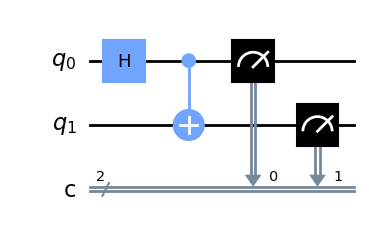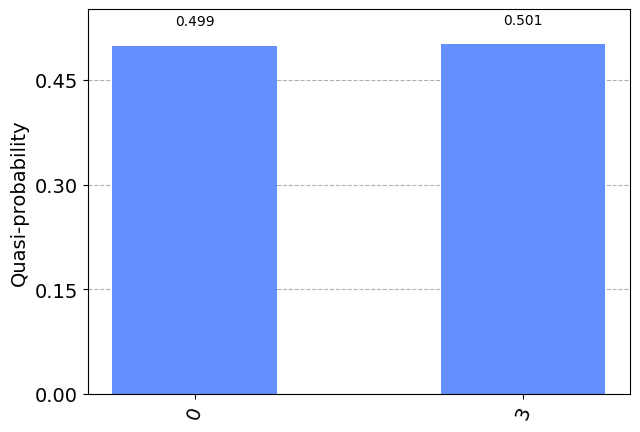# Hello world

Create a simple quantum program and run it on a simulator or real quantum system in this Hello world example. Begin with following the Install and set up instructions if you haven't already, including the steps to Set up to use IBM Quantum Platform.

We recommend that you use the Jupyter (opens in a new tab) development environment to interact with Qiskit. Be sure to install the recommended extra visualization support (`pip install qiskit[visualization]`), and note that zsh users need to put `'qiskit[visualization]'` in single quotes.

To learn about quantum computing in general, check out the Basics of quantum information course (opens in a new tab) in IBM Quantum Learning.

## Write a quantum program

Qiskit uses quantum circuits to represent quantum programs. When creating a circuit, you'll usually create a new `QuantumCircuit` object, then add instructions to it in sequence.

The following code cell creates a circuit that produces a Bell state, which is a specific two-qubit entangled state. For a simple experiment that uses a Bell state to show the effects of entanglement, check out the CHSH game (opens in a new tab).

Note: little-endian bit ordering

Qiskit uses little-endian bit ordering; that is, the bit (or qubit) with index 0 is the least significant qubit. This differs across authors and software packages, so be aware!

 :
``````from qiskit import QuantumCircuit

# Create a new circuit with two qubits (first argument) and two classical
# bits (second argument)
qc = QuantumCircuit(2, 2)

qc.h(0)

# Perform a controlled-X gate on qubit 1, controlled by qubit 0
qc.cx(0, 1)

# Measure qubit 0 to cbit 0, and qubit 1 to cbit 1
qc.measure(0, 0)
qc.measure(1, 1)

# Return a drawing of the circuit using MatPlotLib ("mpl"). This is the
# last line of the cell, so the drawing appears in the cell output.
# Remove the "mpl" argument to get a text drawing.
qc.draw("mpl")``````

Output:See `QuantumCircuit` in the documentation for all available operations.

Quantum circuits can produce random results, so you'll often want to collect a sample of the outputs by running the circuit many times. You can sample a circuit's output using the `Sampler` class. `Sampler` is one of our two `primitives`, which provide methods that make it easier to build modular algorithms and other higher-order programs.

This tutorial uses Qiskit's built-in `Sampler`, which efficiently evaluates the possibility of multiple relevant data points in the context of destructive interference, but can only handle small numbers of qubits. You can replace this with samplers of higher-performance simulators from Qiskit Aer (opens in a new tab), or with samplers that send your circuit over the internet to run on real quantum computers (such as IBM's Qiskit Runtime (opens in a new tab) sampler).

 :
``````from qiskit_ibm_runtime import QiskitRuntimeService, Sampler

service = QiskitRuntimeService()

backend = service.least_busy(simulator=False,operational=True)

# Create a Sampler object
sampler = Sampler(backend)

# Submit the circuit to the sampler
job = sampler.run(qc)

# Once the job is complete, get the result
job.result()``````
Queue times

Queue times on real devices may vary. If you would like to get a faster result on a simulator, consider changing the parameters to `simulator=True` in the code above, or you can replace the `backend =` line with the following instead:

``````# Run on a simulator
backend = service.get_backend("ibmq_qasm_simulator")``````

The `quasi_dists` property is a list of sampled distributions for each circuit we provided. Since we only provided one circuit, the list only has one element.

The distributions are dictionary-like objects. Each key is a measured output, and its value is the fraction of the time that output was measured. In this case, the circuit measurement output was `0` ~50% of the time, and `3` (which is `11` in binary) ~50% of the time.

### Plotting results

You can use the `plot_histogram` function to plot your `Sampler` results. This function returns a MatPlotLib `Figure`, which displays nicely in Jupyter notebooks.

 :
``````from qiskit.visualization import plot_histogram

plot_histogram(
job.result().quasi_dists
)``````

Output:## Next steps

Recommendations
[ ] :
``````import qiskit.tools.jupyter
%qiskit_version_table``````